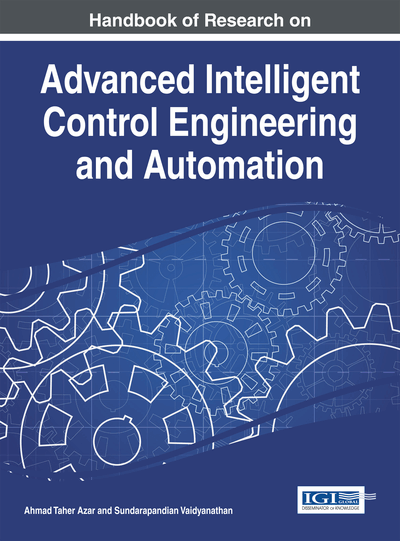# Nonlinear Adaptive Filtering with a Family of Kernel Affine Projection Algorithms

Felix Albu (Valahia University of Targoviste, Romania) and Kiyoshi Nishikawa (Tokyo Metropolitan University, Japan)
Copyright: © 2015 |Pages: 23
DOI: 10.4018/978-1-4666-7248-2.ch002
OnDemand PDF Download:
Available
\$37.50
No Current Special Offers

## Abstract

In this chapter, the family of kernel affine projection algorithms with coherence criterion is presented. The proportionality principle is translated to the kernel-based version. A new algorithm called Kernel Proportionate Affine Projection Algorithm (KPAPA) is proposed. It is proved that the additional computational increase burden is independent of the order of the algorithm, being dependent only on the order of the kernel expansion. The Dichotomous Coordinate Descent (DCD) method and an example of an efficient implementation of KAPA using DCD are presented. This chapter also discusses the influence of the coherence value, the step size value, and the dictionary size on the performance of KAPA and KPAPA algorithms. The effectiveness of the proposed algorithms and the effect of different parameters are confirmed by computer simulations for nonlinear system identification application.
Chapter Preview
Top

## Introduction

Linear adaptive filters have been widely used in a variety of applications, e.g., mobile communications, video processing etc. In system identification applications, the main goal is to identify an unknown system using an adaptive filter. The well known normalized least-mean-square (NLMS) algorithm has been widely used, but converges very slowly (Sayed, 2003). The affine projection algorithm (APA) provides a much improved convergence speed (Sayed, 2003) and in some situations close to that of the more complex recursive least-squares (RLS) algorithm. Another related family of linear algorithms is the proportionate APA (PAPA) family. The update of each coefficient of the filter is made independently of the others, by adjusting the adaptation step-size in proportion to the magnitude of the estimated filter coefficient (Deng, 2006). The proportionate normalized least-mean-square (PNLMS) algorithm (Duttweiler, 2000) was one of the first proportionate-type algorithms. All of the APA/PAPA algorithms require the computation of the inverse of a matrix, which is a complex operation and can lead to algorithm instability if finite precision is used. One of the most efficient methods to avoid the matrix inversion is the use of the Dichotomous Coordinate Descent (DCD) methods (Zakharov & Tozer, 2004), (Zakharov, White & Liu, 2008) and (Albu, Bouchard & Zakharov, 2007).

As an extension of the linear counterparts, kernel adaptive filters have been proposed to identify non-linear systems (Liu, Principe & Haykin, 2010). Kernel adaptive filters are derived by applying the kernel methods to linear adaptive filters, and many such algorithms were proposed, (e.g., the kernel recursive least squares (KRLS) (Engel, Mannor & Meir, 2004), the kernel least mean square (KLMS) (Liu, Pokharel & Principe, 2008), the kernel normalized LMS (KNLMS) (Richard, Bermudez, & Honeine, 2009), kernel affine projection (KAPA) (Richard et al., 2009) and its efficient implementation (Albu, Coltuc, Rotaru & Nishikawa, 2013), etc. The application of a reproducing kernel Hilbert space (RKHS) methodology blended with many signal processing techniques is a powerful tool in solving numerous practical artificial intelligence or control systems problems (Müller, Adali, Fukumizu, Principe, & Theodoridis, 2013).

This chapter explains the similarities between the linear algorithms and their corresponding kernel versions. We start with a short description of the related work and of the linear NLMS, APA, and PAPA algorithms. Next, the kernel AP algorithm with a coherence criterion is presented. Following the resemblance of APA with KAPA, the proportionality criterion is used in order to derive a new algorithm called, kernel PAPA (KPAPA). The KNLMS and a new proportionate version of it are mentioned as particular cases of KAPA and KPAPA respectively. The recent research directions in the development of the reduced complexity algorithms based on DCD iterations are also discussed. Next, the chapter investigates the reduction of the numerical complexity of the kernel algorithms, the fine tuning of the parameters of the algorithms, etc. Their importance and significance for improving the overall performance is discussed. Several simulation results are shown in the Simulation section. Finally, the conclusions and future research direction conclude this work.

## Key Terms in this Chapter

Adaptive Filters: Systems using linear filters whose coefficients adapts according to an optimization algorithm.

Least Mean Square Algorithms: A stochastic gradient descent family of adaptive algorithms that tries to minimize the mean squares of the difference between the desired and the actual signal.

Normalized Least Mean Square Algorithm: A variant of the LMS algorithm whose learning rate is normalized with the power of the input signal.

Dichotomous Coordinate Descend: A multiplier-less and division-less coordinate descent method useful for finding the optimal solution of the normal equations.

Kernel Adaptive Filters: A nonlinear adaptive filter that uses kernel methods.

Proportionate Adaptive Algorithms: Variants of the adaptive algorithms whose coefficients are updated proportionally to the sparsity of the system.

Affine Projection Algorithm: It is a generalization of the NLMS algorithms where the projections are made in multiple dimensions.

## Complete Chapter List

Search this Book:
Reset